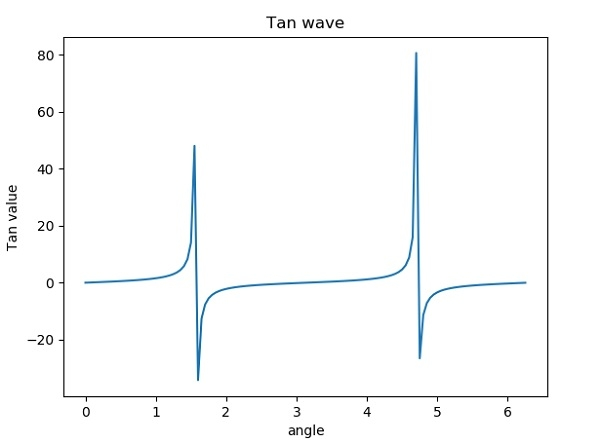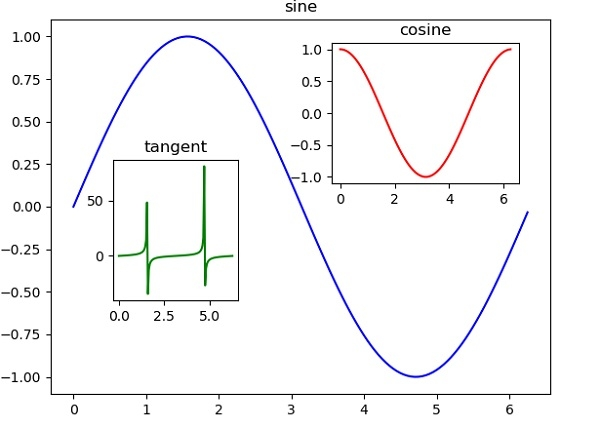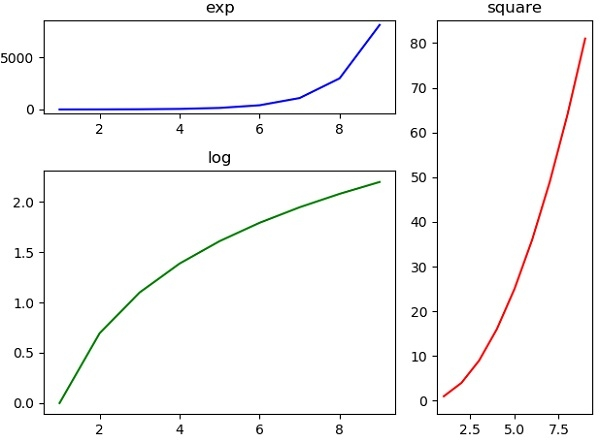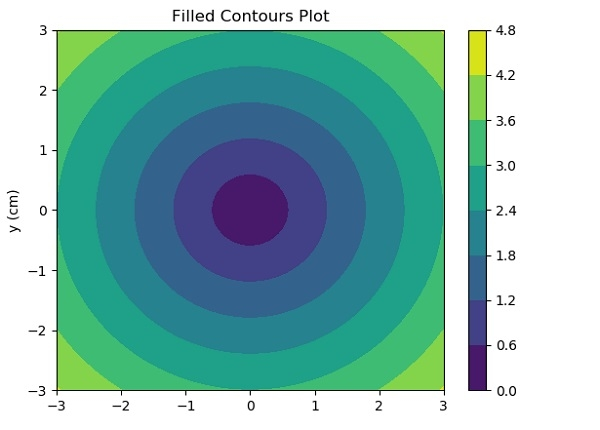# Graph Plotting in Python

Python has the ability to create graphs by using the matplotlib library. It has numerous packages and functions which generate a wide variety of graphs and plots. It is also very simple to use. It along with numpy and other python built-in functions achieves the goal. In this article we will see some of the different kinds of graphs it can generate.

## Simple Graphs

Here we take a mathematical function to generate the x and Y coordinates of the graph. Then we use matplotlib to plot the graph for that function. Here we can apply labels and show the title of the graph as shown below. We are plotting the graph for the trigonometric function − tan.

### Example

from matplotlib import pyplot as plt
import numpy as np
import math #needed for definition of pi
x = np.arange(0, math.pi*2, 0.05)
y = np.tan(x)
plt.plot(x,y)
plt.xlabel("angle")
plt.ylabel("Tan value")
plt.title('Tan wave')
plt.show()

### Output

Running the above code gives us the following result −## Multiplots

We can have two or more plots on a single canvas by creating multiple axes and using them in the program.

### Example

import matplotlib.pyplot as plt
import numpy as np
import math
x = np.arange(0, math.pi*2, 0.05)
fig=plt.figure()
axes1 = fig.add_axes([0.1, 0.1, 0.8, 0.8]) # main axes
axes2 = fig.add_axes([0.55, 0.55, 0.3, 0.3]) # inset axes
axes3 = fig.add_axes([0.2, 0.3, 0.2, 0.3]) # inset axes
axes1.plot(x, np.sin(x), 'b')
axes2.plot(x,np.cos(x),'r')
axes3.plot(x,np.tan(x),'g')
axes1.set_title('sine')
axes2.set_title("cosine")
axes3.set_title("tangent")
plt.show()

### Output

Running the above code gives us the following result −## Grid of Subplots

We can also create a grid containing different graphs each of which is a subplot. For this we use the function subplot2grid. Here we have to choose the axes carefully so that all the subplots can fit in to the grid. A little hit an dtrail may be needed.

### Example

import matplotlib.pyplot as plt
a1 = plt.subplot2grid((3,3),(0,0),colspan = 2)
a2 = plt.subplot2grid((3,3),(0,2), rowspan = 3)
a3 = plt.subplot2grid((3,3),(1,0),rowspan = 2, colspan = 2)
import numpy as np
x = np.arange(1,10)
a2.plot(x, x*x,'r')
a2.set_title('square')
a1.plot(x, np.exp(x),'b')
a1.set_title('exp')
a3.plot(x, np.log(x),'g')
a3.set_title('log')
plt.tight_layout()
plt.show()

### Output

Running the above code gives us the following result:## Contour Plot

Contour plots (sometimes called Level Plots) are a way to show a three-dimensional surface on a two-dimensional plane. It graphs two predictor variables X Y on the y-axis and a response variable Z as contours.Matplotlib contains contour() and contourf() functions that draw contour lines and filled contours, respectively.

### Example

import numpy as np
import matplotlib.pyplot as plt

xlist = np.linspace(-3.0, 3.0, 100)
ylist = np.linspace(-3.0, 3.0, 100)

X, Y = np.meshgrid(xlist, ylist)
Z = np.sqrt(X**2 + Y**2)

fig,ax=plt.subplots(1,1)
cp = ax.contourf(X, Y, Z)
fig.colorbar(cp) # Add a colorbar to a plot
ax.set_title('Filled Contours Plot')

#ax.set_xlabel('x (cm)')
ax.set_ylabel('y (cm)')
plt.show()

### Output

Running the above code gives us the following result: